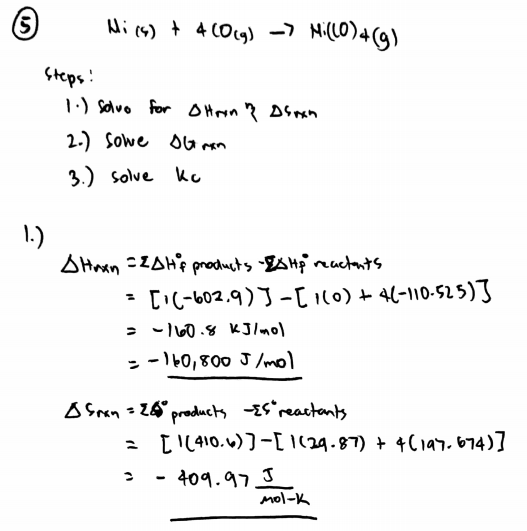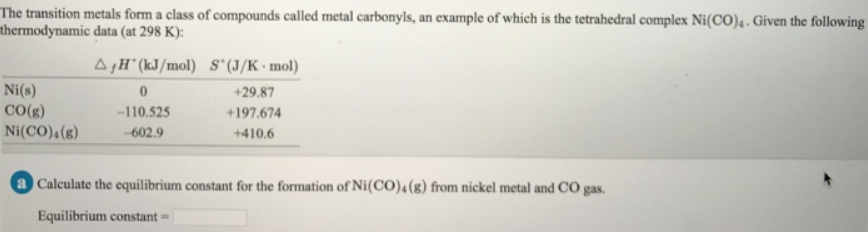# Problem: The transition metals form a class of compounds called metal carbonyls, an example of which is the tetrahedral complex Ni(CO)4. Given the following thermodynamic data (at 298 K): Calculate the equilibrium constant for the formation of Ni(CO) 4 (g) from nickel metal and CO gas.

###### FREE Expert Solution80% (488 ratings)###### Problem Details

The transition metals form a class of compounds called metal carbonyls, an example of which is the tetrahedral complex Ni(CO)4. Given the following thermodynamic data (at 298 K):

Calculate the equilibrium constant for the formation of Ni(CO) 4 (g) from nickel metal and CO gas.